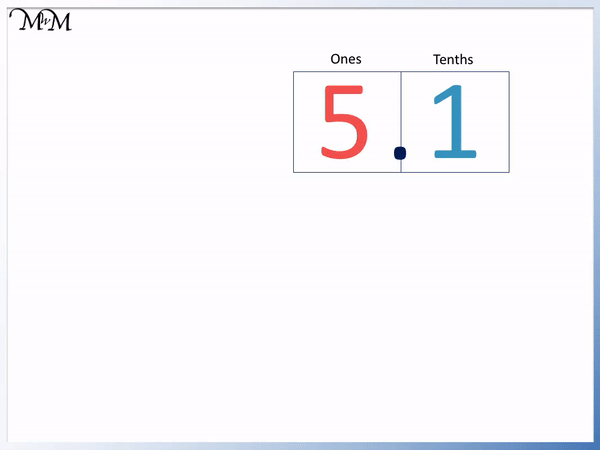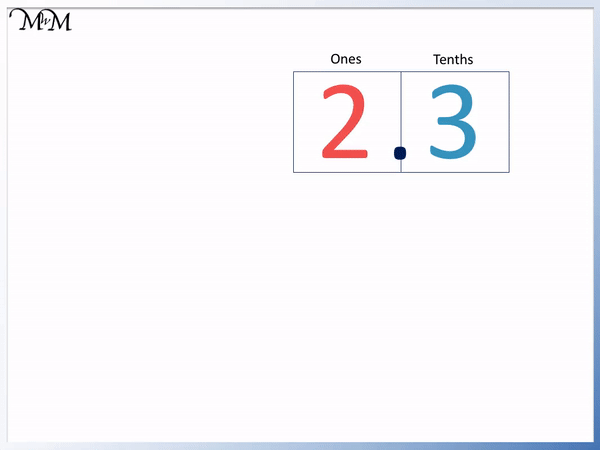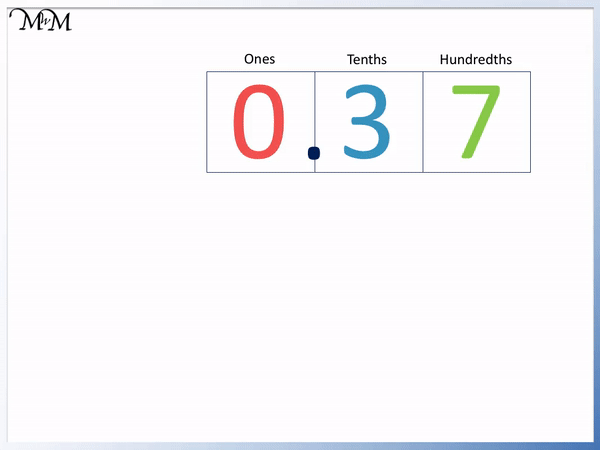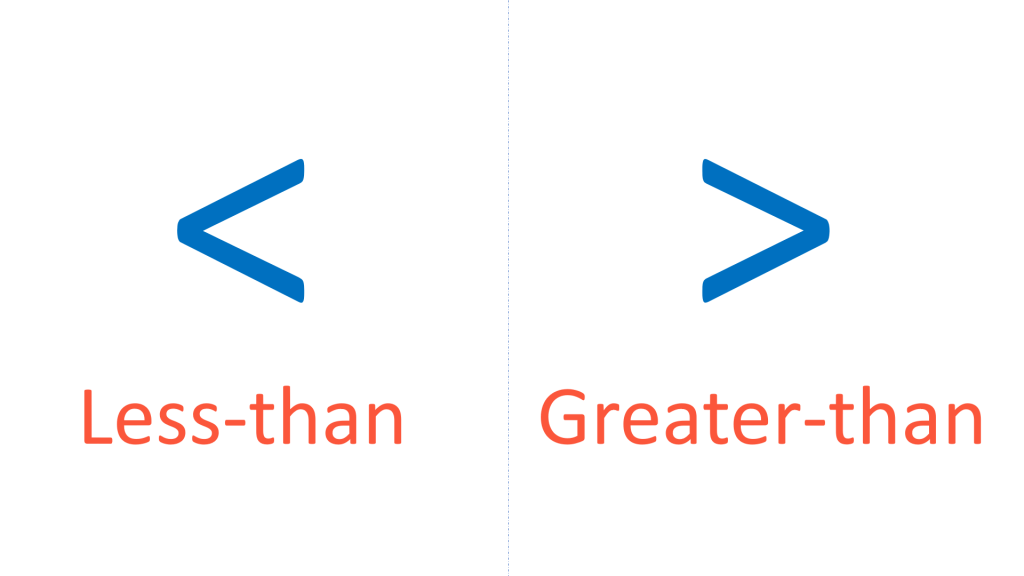# Writing Decimals in Expanded Form

Writing Decimals in Expanded Form• Writing a number in expanded form means to show the value of each non-zero digit in the number.
• The number of non-zero digits tells us how many numbers will be shown in our expanded form answer.
• Digits after the decimal point are worth less than one whole.
• The 1 in the ones column is simply written as 1.
• The 2 is in the tenths column and so, it is worth 2 out of 10.
• 2 tenths is written as a fraction as   2 / 10   .
• The final 1 is in the hundredths column and so, it is worth 1 out of 100.
• 1 hundredth is written as a fraction as   1 / 100  .
• The decimal 1.21 is written in expanded form as 1 +   2 / 10   +   1 / 100  .
• Count the number of places that each digit is to the right of the decimal point to see how many zeros are in the fraction it is out of.

Writing a number in expanded form means to show the value of each digit in the number.

Each decimal digit is written as a fraction.• The digit of 1 before the decimal point is simply worth 1.
• The digits to the right of the decimal point are written as fractions.
• The number of places each digit is to the right of the decimal point is the number of zeros in the of the fraction.
• We don’t write anything for the digit of 0.
• The 8 is in the hundredths column, so we write it as   8 / 100  .
• 8 is two places to the right of the decimal and so, 100 has two zeros.
• The 9 is in the thousandths column and is written as   9 / 1000  .
• The 9 is 3 places to the right of the decimal point and so, 1000 has 3 zeros.
• 1.089 is written in expanded form as 1 +   8 / 100   +   9 / 1000  .# Writing Decimals in Expanded Form

## How to Write Decimals in Expanded Form

The expanded form of a number shows the value of each digit in the number. To write decimals in expanded form write the value of each digit with addition signs between them.

Digits to the left of the decimal point are whole numbers. Digits to the right of the decimal point are less than one and are written as fractions.

The number of places each digit is to the right of the decimal point is the same as the number of zeros in the

of the fraction.

For example, we will write the decimal number 5.1 in expanded form.

5 is in the one column and so is just worth 5.

The 1 is in the tenths column and so is worth 1 tenth.1 tenth is written as   1 / 10  .

The digit at the top of the fraction is the digit we are looking at, which is 1.

The digit 1 is one place to the right of the decimal point and so the number on the bottom of the fraction contains 1 zero.

We can write the decimal 5.1 in expanded form as 5 +   1 / 10  .

Here is another example writing decimals in the expanded form. We will look at 2.3.

2 is in the ones column and so, it is just worth 2.

The 3 is in the tenths column and so, it is worth 3 tenths.3 tenths is written as   3 / 10  .

The digit on the top of the fraction is the digit we are looking at, which is 3. This digit is one place right of the decimal point and so, the number on the bottom of the fraction contains one zero.

The decimal number 2.3 is written in expanded form as 2 +   3 / 10  .

The next example is 1.21. We will write this decimal in expanded form.

1 is in the ones column and so, it is just worth 1.

The 2 is in the tenths column and so, it is written as   2 / 10  .

The final 1 is in the hundredths column and so, it is worth 1 hundredth.1 hundredth is written as   1 / 100  .

The digit on the top of the fraction is the digit we are looking at, which is 1. This digit is two places to the right of the decimal point and so the number on the bottom of the fraction contains two zeros.

The decimal 1.21 is written in expanded form as 1 +   2 / 10   +   1 / 100  .

The next example of writing decimals in expanded form is 0.37.

Remember that we only write the values of non-zero digits. We ignore the 0 in the ones column because it is not worth anything.

The 3 is in the tenths column and so it is worth 3 tenths,   3 / 10  .

The 7 is in the hundredths column and so it is worth 7 hundredths,   7 / 100  .We can write 0.37 in expanded form as   3 / 10   +   7 / 100  .

Notice that we only have two non-zero digits in 0.37: 3 and 7. Therefore we only have two numbers in our expanded form answer.

Here is our next example of writing 4.231 in expanded form.

4 is in the ones column and is worth 4.

2 is in the tenths column and is worth 2 tenths,   2 / 10  .

3 is in the hundredths column and is worth 3 hundredths,   3 / 100  .

The 1 is in the thousandths column and is worth 1 thousandth,   1 / 1000  .The 1 is three places to the right of the decimal point and so the fraction   1 / 1000   contains 3 zeros on the bottom.

The decimal number 4.231 is written in expanded form as 4 +   2 / 10   +   3 / 100   +   1 / 1000  .

In the next example we write 0.503 in expanded form.

We only consider the digits that are not zero.

We have 5 tenths, which is written as   5 / 10  .

We have 3 thousandths, which is written as   3 / 1000  .0.503 is written in expanded form as   5 / 10   +   3 / 1000  .

In the next example we write the decimal 1.089 in expanded form.

The 1 is in the ones column and is simply written as 1.

We ignore the zero.

The 8 is in the hundredths column and is written as   8 / 100  .

The 9 is in the thousandths column and is written as   9 / 1000  .1.089 is written in expanded form as 1 +   8 / 100   +   9 / 1000  .

There are 3 non-zero digits in 1.089: 1, 8 and 9.